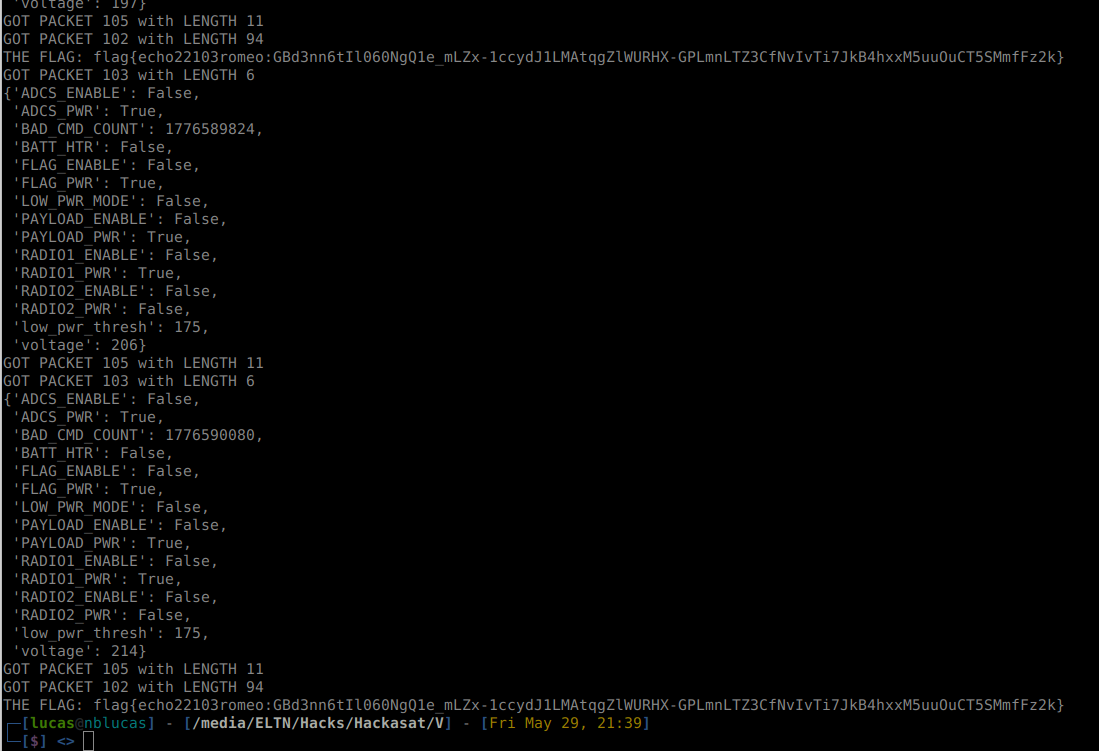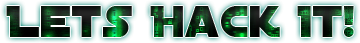Hack-a-sat — Can you hear me now?

That challenged asked us to decode a Telemetry data that was being sent over a TCP port. If you open the netcat, the following happen: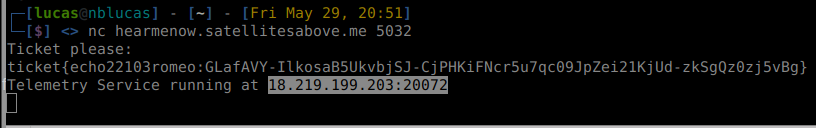Then if you connect to the Telemetry Service using netcat: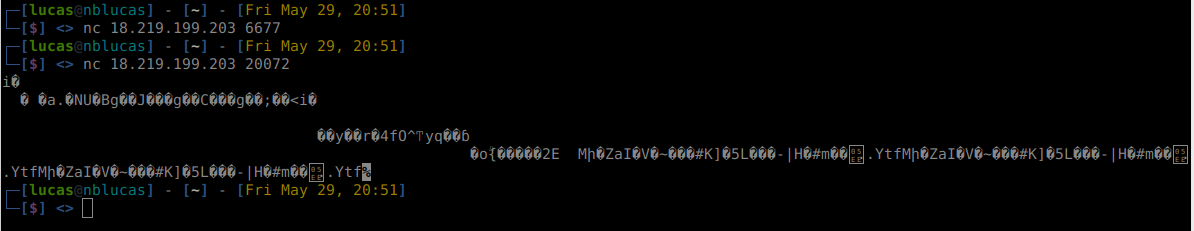In the provided zip file there is a telemetry.xtce file which is a XML file that tells us how the binary packet is encoded. A quick search over the internet lead me to the Wikipedia: https://en.wikipedia.org/wiki/XML_Telemetric_and_Command_Exchange

It is defined in the CCSDS Green Book (the spec https://public.ccsds.org/Pubs/660x0g1.pdf )

The file has several sections. I will describe a few of them:

• ParameterTypeSet — Describes the type of parameters to be used in the protocol definition
• ParameterSet — Describes parameters to be used in protocol definition
• ContainerSet — Describes the structures inside the protocol

We will get back to them afterwards. I’m bit lazy to check the whole spec since it should be straightforward to read directly the XTCE. I usually find that when it comes to Aerospace Stuff (Sorry NASA and friends) it’s easier to reverse engineer than read the docs. So I just did a quick look in the documentation and quit (its huge).

By a quick look inside the xtce file we can find how the flag is defined: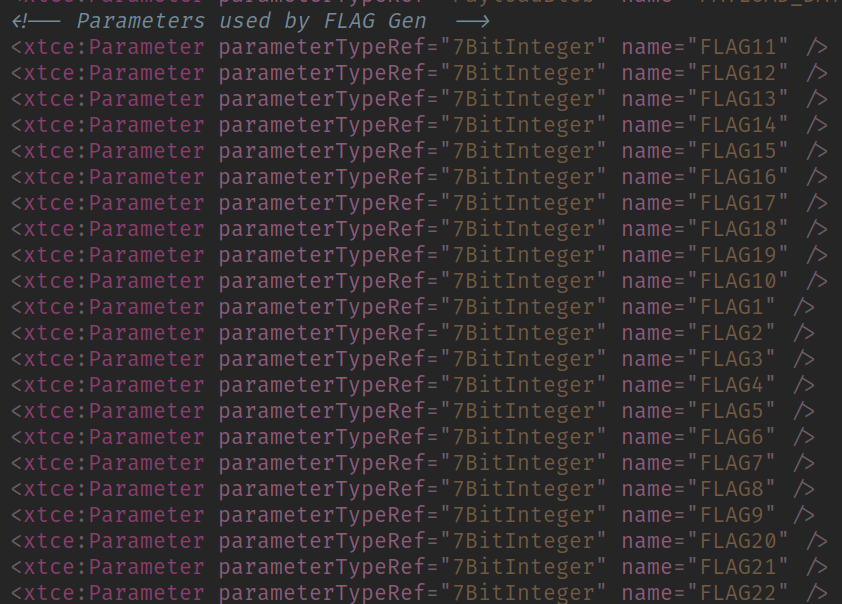Each of the parameters looks like a single character from the flag, but they’re 7 bit encoded. That means if we look at the raw binary, we will not see the flag itself since each ASCII character is 8 bits wide.

In the ContainerSet we can see the possible structs that we received. Let’s take a look in a piece: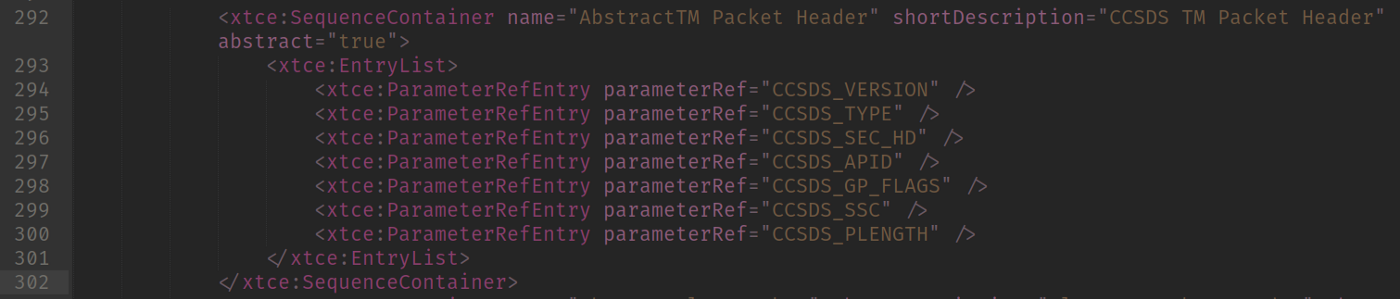AbstractTM Packet Header Definition

That piece specifies a Container called “AbstractTM Packet Header” (I think I will launch a company called Abstract after that) in which there are few entries. These entries are mapped to the binary itself. Notice the parameterRef which points to a previous defined parameter at ParameterSet section. Let’s take a look: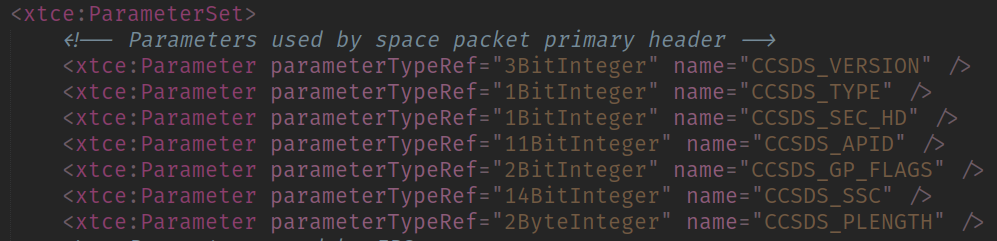CCSDS Header Parameter Types

From there we can infer:

• The field CCSDS_VERSION is encoded in 3 bit
• The field CCSDS_TYPE is encoded in 1 bit
• The field CCSDS_SEC_HD is encoded in 1 bit
• The field CCSDS_APID is encoded in 11 bit
• The field CCSDS_GP_FLAGS is encoded in 2 bit
• The field CCSDS_SSC is encoded in 14 bit
• The field CCSDS_PLENGTH is encoded in 2 bytes (16 bit)

If you sum up all, you will get a header that is 6 bytes long. When seeing this two fields came to my attention. The APID and PLENGTH . APID is usually refered to APplication IDentification and PLENGTH to Packet LENGTH.

That means that even if there are a lot of packets, we dont need to really parse them, just the flag one. We can skip by knowing the APID and Packet Length. So let’s search the Flag packet!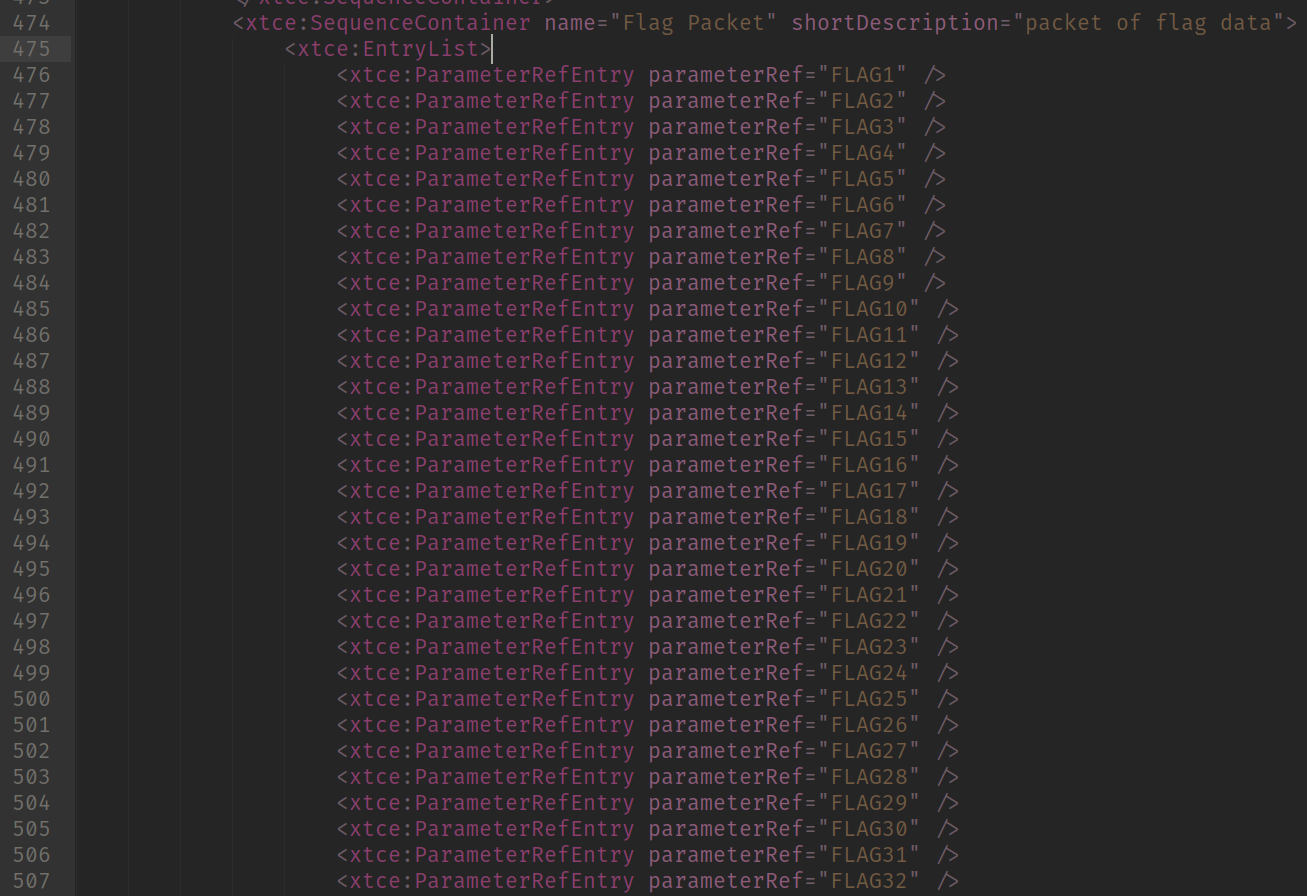Start of Flag Packet definition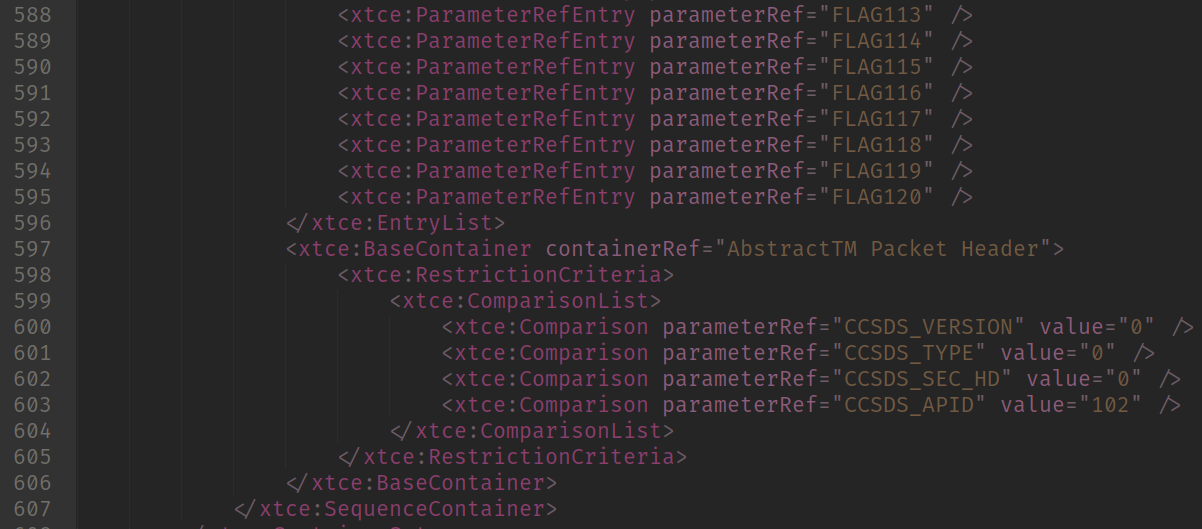End of Flag Packet definition

So here I split the image because the packet definition is huge (lots of FLAGXX fields). We can see a new section here: RestrictionCriteria.

That section tells us which is the condition that the parser should met to parse the content as that packet. That says that if a packet has the following Field => Values in the Packet Header:

• CCSDS_VERSION => 0
• CCSDS_TYPE => 0
• CCSDS_SEC_HD => 0
• CCSDS_APID => 102

That means we got our Flag Packet. So let’s assume all packets have a header and make our parser!

I started dumping the netcat to a file so I can process without having to connect every time. That’s simple by just piping:

nc 18.219.199.203 20072 > dump.bin

Then started writing a simple python script. First by

import struct

f = open("dump.bin", "rb")
f.close()

c_version = (data & 0xE0) >> 5
c_type = (data & 0x10) >> 4
c_sechd = (data & 0x8) >> 3
c_apid = ((data & 0x7) << 8) + data
c_gpflags = (data & 0xC0) >> 6
c_ssc = ((data & 0x3F) << 8) + data
c_plen = (data<< 8) + (data )
return c_version, c_type, c_sechd, c_apid, c_gpflags, c_ssc, c_plen

while len(data) > 0:
c_version, c_type, c_sechd, c_apid, c_gpflags, c_ssc, c_plen = DecodeHeader(data)
print("GOT PACKET %d with LENGTH %d" %(c_apid, c_plen))
data = data[6:]         # Skip the header
# data[:c_plen+1] has content
data = data[c_plen+1:]  # remove the current data

This decodes the header by doing some bit shifting. And if my assumption that every packet has a header and the packet length I could iterate over the file until no bytes are left. Since the file is small, I could load the entire file in the memory. Then running the script gave me: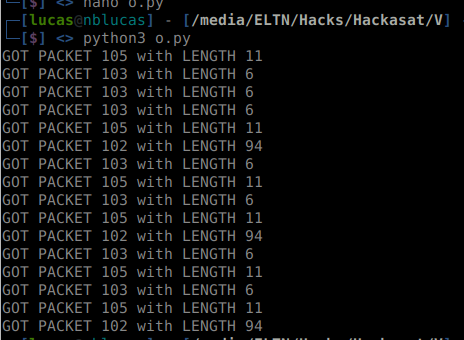Got no errors and the data looks fine! We also got the APID 102 which is what the APID for Flag Packet. Then the content should be easy to read.

If we check the Flag Packet definition, besides the header, there is only the FLAGXXX fields there which means the entire content is the flag. Then we can just get the whole content and decode from 7 bit to 8 bit. Do do that, I was really lazy to do the proper bit shifting, so I just created an array with 1’s and 0’s strings, when it reached 8, I packed into a char and added to an array. That would be really slow for big data, but for a 120 byte flag should be good. So thats the code I tried:

import struct

f = open("dump.bin", "rb")
f.close()

c_version = (data & 0xE0) >> 5
c_type = (data & 0x10) >> 4
c_sechd = (data & 0x8) >> 3
c_apid = ((data & 0x7) << 8) + data
c_gpflags = (data & 0xC0) >> 6
c_ssc = ((data & 0x3F) << 8) + data
c_plen = (data<< 8) + (data )
return c_version, c_type, c_sechd, c_apid, c_gpflags, c_ssc, c_plen

def DecodeFlag(data):
bitstream = []
for i in range(len(data)):
for b in format(data[i], "08b"):
bitstream.append(b)

flagdata = ""

while len(bitstream) > 0:
b = ""
for i in range(7):
if len(bitstream) > i:
b += bitstream[i]

if b != "":
flagdata += chr(int(b, 2))
bitstream = bitstream[7:]

return flagdata

while len(data) > 0:
c_version, c_type, c_sechd, c_apid, c_gpflags, c_ssc, c_plen = DecodeHeader(data)
print("GOT PACKET %d with LENGTH %d" %(c_apid, c_plen))
data = data[6:]         # Skip the header
# data[:c_plen+1] has content
if c_apid == 102:
flag = DecodeFlag(data[:c_plen+1])
print("THE FLAG: %s" % flag)
data = data[c_plen+1:]  # remove the current data

Then when running: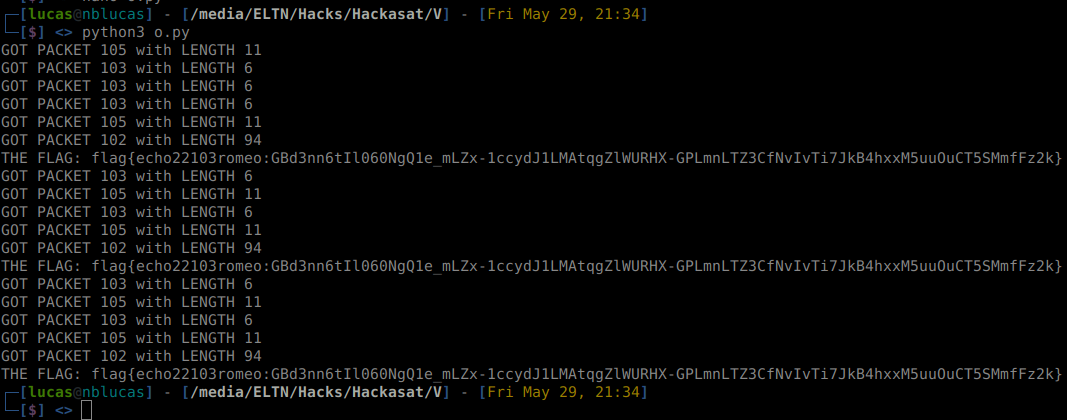BINGO, THERE IS OUR FLAG!

flag{echo22103romeo:GBd3nn6tIl060NgQ1e_mLZx-1ccydJ1LMAtqgZlWURHX-GPLmnLTZ3CfNvIvTi7JkB4hxxM5uuOuCT5SMmfFz2k}

I hope you liked the explanation. I didn’t take a deep dive in XTCE stuff but just the enough to get the flag. The XTCE format looks interesting (pretty much like a protobuf but in XML) and I will take a look eventually. That also has been my first CTF in my life and was really fun to play!

BONUS

We can also decode the EPS data which should give us some satellite info (and a spoiler to the next flag):

import struct

from pprint import pprint

f = open("dump.bin", "rb")
f.close()

c_version = (data & 0xE0) >> 5
c_type = (data & 0x10) >> 4
c_sechd = (data & 0x8) >> 3
c_apid = ((data & 0x7) << 8) + data
c_gpflags = (data & 0xC0) >> 6
c_ssc = ((data & 0x3F) << 8) + data
c_plen = (data<< 8) + (data )
return c_version, c_type, c_sechd, c_apid, c_gpflags, c_ssc, c_plen

def DecodeFlag(data):
bitstream = []
for i in range(len(data)):
for b in format(data[i], "08b"):
bitstream.append(b)

flagdata = ""

while len(bitstream) > 0:
b = ""
for i in range(7):
if len(bitstream) > i:
b += bitstream[i]

if b != "":
flagdata += chr(int(b, 2))
bitstream = bitstream[7:]

return flagdata

def DecodeEPS(data):
battemp = data[0:2]
voltage = (struct.unpack(">H", data[2:4]))
low_pwr_thresh = (struct.unpack(">H", data[4:6]))
data = data[6:]

LOW_PWR_MODE  = (data & (1 << 0)) > 0
BATT_HTR      = (data & (1 << 1)) > 0
PAYLOAD_PWR   = (data & (1 << 2)) > 0
FLAG_PWR      = (data & (1 << 3)) > 0
ADCS_PWR      = (data & (1 << 4)) > 0
RADIO1_PWR    = (data & (1 << 5)) > 0
RADIO2_PWR    = (data & (1 << 6)) > 0

data = data[1:]

PAYLOAD_ENABLE = (data & (1 << 0)) > 0
FLAG_ENABLE    = (data & (1 << 1)) > 0
ADCS_ENABLE    = (data & (1 << 2)) > 0
RADIO1_ENABLE  = (data & (1 << 3)) > 0
RADIO2_ENABLE  = (data & (1 << 4)) > 0

data = data[1:]

return {
"low_pwr_thresh": low_pwr_thresh,
"voltage": voltage,
"LOW_PWR_MODE": LOW_PWR_MODE,
"BATT_HTR": BATT_HTR,
"FLAG_PWR": FLAG_PWR,
"FLAG_ENABLE": FLAG_ENABLE,
}

while len(data) > 0:
c_version, c_type, c_sechd, c_apid, c_gpflags, c_ssc, c_plen = DecodeHeader(data)
print("GOT PACKET %d with LENGTH %d" %(c_apid, c_plen))
data = data[6:]         # Skip the header
# data[:c_plen+1] has content
if c_apid == 102:
flag = DecodeFlag(data[:c_plen+1])
print("THE FLAG: %s" % flag)
elif c_apid == 103:
pprint(DecodeEPS(data))
data = data[c_plen+1:]  # remove the current data